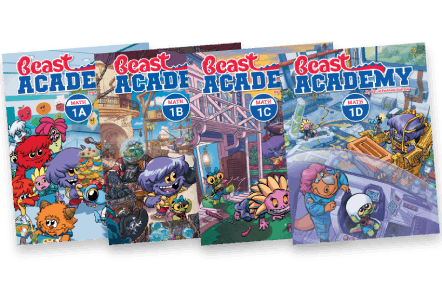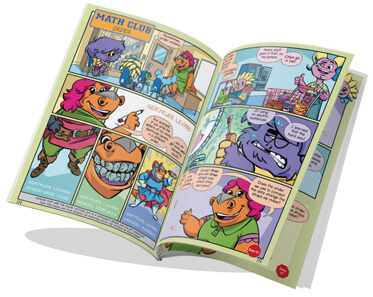By Jason Batterson
Illustrated by Erich Owen

# Level 1 Math Books

Ages 6‑8By Jason Batterson
Illustrated by Erich Owen## Level 1 Books Information

Our Level 1 curriculum includes four books, one for each unit (1A, 1B, 1C, 1D). These books include Guide sections written in an engaging comic book style, and over 400 practice problems ranging from introductory level exercises to very challenging puzzles and word problems.

Please note that, unlike for Levels 2 and above, Level 1 does not have separate Guide and Practice books. There is only one book per unit.Level 1 has four units: A, B, C and D. Topics build upon each other from unit to unit.

1A
1B
1C
1D
Counting
Keeping track, recognizing small amounts (subitizing), grouping, ten frames, hundreds charts.
Symbols (+ and =), writing addition, finding a missing addend, pairs with the same sum (number bonds), adding more than two numbers, making ten, order (commutativity), and counting up.
Strategies review, using the number line, distance on the number line, adding and subtracting tens, adding by place value.
Big Numbers
Reading and writing numbers from 100 to 999, counting by tens, block models of place value, regrouping, and expanded form.
Shapes
Names and properties, rotations and reflections, combinations and dissections, polyominoes.
Subtraction
Taking away, subtracting 10, counting down, the relationship between addition and subtraction, finding a difference, ordering, and strategies.
Comparing
Ordering numbers using place value, comparing expressions, finding sums and differences that are equal, compensation strategies.
Measurement
Comparing lengths, using units, using a ruler, units of time, and reading analog and digital clocks.
Comparing
Equal amounts, matching (correspondence), symbols (=, <, and >), ordering within 100, comparing length and distance.
Categories
Properties, finding similarities and differences, which doesn't belong, categories of numbers (odds, evens, squares, and primes), Venn diagrams.
Patterns
Completing patterns that use shapes and numbers, skip-counting, and special patterns including the Fibonacci sequence, perfect squares, and triangular numbers.
Problem Solving
Understanding directions, describing position, rows and columns, and recognizing order.

We recommend taking the Level 1A Placement Test to determine if your student is ready to take Beast Academy Level 1.

Solutions to the placement test are found at the end of the test. Your student may also take the Level 1B, 1C, or 1D placement tests to determine readiness per unit.I'm pretty sure they are the most engaging math books I've ever encountered, and they pull this off without watering down the content at all (quite the contrary, in fact).

Jessie Mathisen, Elementary School Teacher